# FAQ of PUNIAS

Last updated Aug. 21th, 2019

### Answers about PUNIAS

• Is PUNIAS working under a Mac OS? [top]
• PUNIAS is written in C++ using OpenGl for the graphics and MFC (Microsoft Fundation Classes) for the entire GUI (Graphical User Interface).
The last part of the code can't directly be exported on a Mac Os,

• All AFM facilities are working on PC computers explaining why we only wrote PUNIAS under a PC.

• There are so many analysis methods within Punias that I'm kind of lost and don't know which one I should use in order to analyse my expriment.
Which of the following analysis methods should I use?:
[top]
• - Protein Unfolding [top]
This analysis method can be used in order to analyse protein unfolding type of experiments in order to extract the unfolding lengths and forces of unfolding events, the loading rates of lingand/receptor interactions, the overall and single event adhesion energies and the hysteresis energy between the extension and retraction curves.

The data obtained in the generated results file are the following:
• file: Name of the experimental file being analyzed.

• peak (nm): Position in nanometer of the chosen peaks in relative unit.

• height (nm): Height of the peaks in nanometer, corresponding to the difference between the deflection of the given peak and the baseline.

• force (pN): Force of the peaks in Pico Newton, corresponding to the product between the height and the spring constant.

• detachment work (pN): Detachment work of the peaks in Pico Newton corresponding to the distance in force between the beginning and the end of the unfolding peaks.

• unfolding length (nm): Unfolding length in nanometers of a protein domain, corresponding to the difference between two consecutive peak positions. The unfolding length is calculated with the difference of the peak position minus the difference of height, taking thus into account the deflection of the cantilever. The first value corresponds to the length measured between the first unfolding peak and the intersection between the experimental curve and the baseline.

• loading rate (pN/s): Loading rate in Pico Newton per second corresponding to the system elasticity (i.e. the slope of the given force versus deformation unfolding peak) times the retract velocity.

• loading rate 2nd order(pN/s): Loading rate in Pico Newton per second obtained by using a second order polynomial fit regression.

• single event unfolding work (pN.nm): Unfolding work in Pico Newton nanometers of one unfolding event, corresponding to the area found between the height defined by the left marker and the unfolding curve.

• total unfolding length (nm): Total unfolding length in nanometers, corresponding to the sum of all the previous calculated lengths minus the unfolding length between the two first peaks. This length corresponds to the total length the protein was pulled off the surface.

• tip velocity (nm/s): Tip velocity in nanometer per second during an unbinding process.

• curves difference area (pN.nm): Curves difference area in Pico Newton nanometers, corresponding to the area found between the extension and retraction curve.

• unfolding work (pN.nm): Unfolding work in Pico Newton nanometers, corresponding to the area found between the baseline and the unfolding curve.

• involvs (nm/V): Involvs (INVerse Optical LeVer Sensitivity) calibration of the cantilever in nanometer per Volt.

• spring constant (pN/nm): Spring constant value of the cantilever in pico-Newton per nanometer.

• index of baseline (-): Index position of the point used for the baseline.

• index of origin (-): Index position of the point used for the origin of the curve.

• index of area (-): Index position of the first marker used for the adhesion energy analysis.

• index of difference (-): Index position of the first marker used for the curve difference area analysis.

A list of the short keys can be found under the Help Menu of Short Keys command.

- Protein Unfolding WLCM [top]
This analysis method can be used in order to analyse protein unfolding type of experiments with WLCM (Worm Like Chain Model) fits.
The equation used for performing the curve fitting is the following:with:
 - F the force, - x the chain extension, - p the persistence length, - L the contour length, - kB the Boltzmann constant, - T the absolute temperature.

The data obtained in the generated results file are the following:
• file: Name of the experimental file being analyzed.

• persistence length (nm): Persistence length in nanometer of the chosen unfolding peak.

• contour length (nm): Contour length in nanometer of the chosen unfolding peak.

• R^2 (-): Coefficient of determination obtained for the WLCM fit by scanning through the data points between the chosen markers.

• extension 1 (nm): Extension in nanometer corresponding to the position of the first cursor used for delimiting the data points used for the fitting procedure.

• extension 2 (nm): Extension in nanometer corresponding to the position of the second cursor used for delimiting the data points used for the fitting procedure.

• height 1 (nm): Height in nanometer of the first cursor used for used for delimiting the data points used for the fitting procedure.

• height 2 (nm): Height in nanometer of the second cursor used for used for delimiting the data points used for the fitting procedure.

• force 1 (pN): Force in pico-Newton of the first cursor used for used for delimiting the data points used for the fitting procedure.

• force 2 (pN): Force in pico-Newton of the second cursor used for delimiting the data points used for the fitting procedure.

• detachment work (pN): Detachment work of the peaks in Pico Newton corresponding to the distance in force between the beginning and the end of the unfolding peaks.

• unfolding length (nm): Unfolding length in nanometers of a protein domain, corresponding to the difference between two consecutive peak positions. The unfolding length is calculated with the difference of the peak position minus the difference of height, taking thus into account the deflection of the cantilever. The first value corresponds to the length measured between the first unfolding peak and the intersection between the experimental curve and the baseline.

• loading rate (pN/s): Loading rate in Pico Newton per second corresponding to the system elasticity (i.e. the slope of the given force versus deformation unfolding peak) times the retract velocity.

• loading rate 2nd order(pN/s): Loading rate in Pico Newton per second obtained by using a second order polynomial fit regression.

• single event unfolding work (pN.nm): Unfolding work in Pico Newton nanometers of one unfolding event, corresponding to the area found between the height defined by the left marker and the unfolding curve.

• total unfolding length (nm): Total unfolding length in nanometers, corresponding to the sum of all the previous calculated lengths minus the unfolding length between the two first peaks. This length corresponds to the total length the protein was pulled off the surface.

• tip velocity (nm/s): Tip velocity in nanometer per second during an unbinding process.

• curves difference area (pN.nm): Curves difference area in Pico Newton nanometers, corresponding to the area found between the extension and retraction curve.

• unfolding work (pN.nm): Unfolding work in Pico Newton nanometers, corresponding to the area found between the baseline and the unfolding curve.

• involvs (nm/V): Involvs (INVerse Optical LeVer Sensitivity) calibration of the cantilever in nanometer per Volt.

• spring constant (pN/nm): Spring constant value of the cantilever in pico-Newton per nanometer.

• index of baseline (-): Index position of the point used for the baseline.

• index of origin (-): Index position of the point used for the origin of the curve.

• index of area (-): Index position of the first marker used for the adhesion energy analysis.

• index of difference (-): Index position of the first marker used for the curve difference area analysis.

A list of the short keys can be found under the Help Menu of Short Keys command.

- Protein Unfolding FJCM [top]
This analysis method can be used in order to analyse protein unfolding type of experiments with FJCM (Freely Jointed Chain Model) fits.
The equation used for performing the curve fitting is the following:with:
 - F the force, - x the chain extension, - a the Kuhn length, - L the contour length, - kB the Boltzmann constant, - T the absolute temperature.

The data obtained in the generated results file are the following:
• file: Name of the experimental file being analyzed.

• Kuhn length (nm): Kuhn length in nanometer of the chosen unfolding peak.

• contour length (nm): Contour length in nanometer of the chosen unfolding peak.

• R^2 (-): Coefficient of determination obtained for the WLCM fit by scanning through the data points between the chosen markers.

• extension 1 (nm): Extension in nanometer corresponding to the position of the first cursor used for delimiting the data points used for the fitting procedure.

• extension 2 (nm): Extension in nanometer corresponding to the position of the second cursor used for delimiting the data points used for the fitting procedure.

• height 1 (nm): Height in nanometer of the first cursor used for used for delimiting the data points used for the fitting procedure.

• height 2 (nm): Height in nanometer of the second cursor used for used for delimiting the data points used for the fitting procedure.

• force 1 (pN): Force in pico-Newton of the first cursor used for used for delimiting the data points used for the fitting procedure.

• force 2 (pN): Force in pico-Newton of the second cursor used for

• detachment work (pN): Detachment work of the peaks in Pico Newton corresponding to the distance in force between the beginning and the end of the unfolding peaks.

• unfolding length (nm): Unfolding length in nanometers of a protein domain, corresponding to the difference between two consecutive peak positions. The unfolding length is calculated with the difference of the peak position minus the difference of height, taking thus into account the deflection of the cantilever. The first value corresponds to the length measured between the first unfolding peak and the intersection between the experimental curve and the baseline.

• loading rate (pN/s): Loading rate in Pico Newton per second corresponding to the system elasticity (i.e. the slope of the given force versus deformation unfolding peak) times the retract velocity.

• loading rate 2nd order(pN/s): Loading rate in Pico Newton per second obtained by using a second order polynomial fit regression.

• single event unfolding work (pN.nm): Unfolding work in Pico Newton nanometers of one unfolding event, corresponding to the area found between the height defined by the left marker and the unfolding curve.

• total unfolding length (nm): Total unfolding length in nanometers, corresponding to the sum of all the previous calculated lengths minus the unfolding length between the two first peaks. This length corresponds to the total length the protein was pulled off the surface.

• tip velocity (nm/s): Tip velocity in nanometer per second during an unbinding process.

• curves difference area (pN.nm): Curves difference area in Pico Newton nanometers, corresponding to the area found between the extension and retraction curve.

• unfolding work (pN.nm): Unfolding work in Pico Newton nanometers, corresponding to the area found between the baseline and the unfolding curve.

• involvs (nm/V): Involvs (INVerse Optical LeVer Sensitivity) calibration of the cantilever in nanometer per Volt.

• spring constant (pN/nm): Spring constant value of the cantilever in pico-Newton per nanometer.

• index of baseline (-): Index position of the point used for the baseline.

• index of origin (-): Index position of the point used for the origin of the curve.

• index of area (-): Index position of the first marker used for the adhesion energy analysis.

• index of difference (-): Index position of the first marker used for the curve difference area analysis.

A list of the short keys can be found under the Help Menu of Short Keys command.

- Protein unfolding FRCM [top]
This analysis method can be used in order to analyse protein unfolding type of experiments with FRCM (Freely Rotating Chain Model) fits.
The equation used for performing the curve fitting is the following: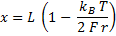or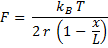with:
 - F the force, - x the chain extension, - r the rotating unit length, - L the contour length, - kB the Boltzmann constant, - T the absolute temperature.

The data obtained in the generated results file are the following:
• file: Name of the experimental file being analyzed.

• rotating unit length (nm): Rotating unit length in nanometer of the chosen unfolding peak.

• contour length (nm): Contour length in nanometer of the chosen unfolding peak.

• R^2 (-): Coefficient of determination obtained for the WLCM fit by scanning through the data points between the chosen markers.

• deformation 1 (nm): Deformation in nanometer corresponding to the position of the first cursor used for delimiting the data points used for the fitting procedure.

• deformation 2 (nm): Deformation in nanometer corresponding to the position of the second cursor used for delimiting the data points used for the fitting procedure.

• height 1 (nm): Height in nanometer of the first cursor used for used for delimiting the data points used for the fitting procedure.

• height 2 (nm): Height in nanometer of the second cursor used for used for delimiting the data points used for the fitting procedure.

• force 1 (pN): Force in pico-Newton of the first cursor used for used for delimiting the data points used for the fitting procedure.

• force 2 (pN): Force in pico-Newton of the second cursor used for

• detachment work (pN): Detachment work of the peaks in Pico Newton corresponding to the distance in force between the beginning and the end of the unfolding peaks.

• unfolding length (nm): Unfolding length in nanometers of a protein domain, corresponding to the difference between two consecutive peak positions. The unfolding length is calculated with the difference of the peak position minus the difference of height, taking thus into account the deflection of the cantilever. The first value corresponds to the length measured between the first unfolding peak and the intersection between the experimental curve and the baseline.

• loading rate (pN/s): Loading rate in Pico Newton per second corresponding to the system elasticity (i.e. the slope of the given force versus deformation unfolding peak) times the retract velocity.

• loading rate 2nd order(pN/s): Loading rate in Pico Newton per second obtained by using a second order polynomial fit regression.

• single event unfolding work (pN.nm): Unfolding work in Pico Newton nanometers of one unfolding event, corresponding to the area found between the height defined by the left marker and the unfolding curve.

• total unfolding length (nm): Total unfolding length in nanometers, corresponding to the sum of all the previous calculated lengths minus the unfolding length between the two first peaks. This length corresponds to the total length the protein was pulled off the surface.

• tip velocity (nm/s): Tip velocity in nanometer per second during an unbinding process.

• curves difference area (pN.nm): Curves difference area in Pico Newton nanometers, corresponding to the area found between the extension and retraction curve.

• unfolding work (pN.nm): Unfolding work in Pico Newton nanometers, corresponding to the area found between the baseline and the unfolding curve.

• involvs (nm/V): Involvs (INVerse Optical LeVer Sensitivity) calibration of the cantilever in nanometer per Volt.

• spring constant (pN/nm): Spring constant value of the cantilever in pico-Newton per nanometer.

• index of baseline (-): Index position of the point used for the baseline.

• index of origin (-): Index position of the point used for the origin of the curve.

A list of the short keys can be found under the Help Menu of Short Keys command.

- Force Steps [top]
This analysis method can be used in order to analyse force steps type of experiments in order to extract the steps forces or distances.

The data obtained in the generated results file are the following:
• file: Name of the experimental file being analyzed.

• dx (nm): Difference of position in nanometer between the cursors.

• dy (pN): Difference of height in pico-Newton between the cursors.

• deformation 1 (nm): Deformation in nanometer corresponding to the position of the first cursor.

• deformation 2 (nm): Deformation in nanometer corresponding to the position of the second cursor.

• height 1 (nm): Height in nanometer of the first cursor.

• height 2 (nm): Height in nanometer of the second cursor.

• force 1 (pN): Force in pico-Newton of the first cursor.

• force 2 (pN): Force in pico-Newton of the second cursor.

• involvs (nm/V): Involvs (INVerse Optical LeVer Sensitivity) calibration of the cantilever in nanometer per Volt.

• spring constant (pN/nm): Spring constant value of the cantilever in pico-Newton per nanometer.

• index of baseline (-): Index position of the point used for the baseline.

• index of origin (-): Index position of the point used for the origin of the curve.

A list of the short keys can be found under the Help Menu of Short Keys command.

- Surface Breakthrough [top]
This analysis method can be used in order to analyse surface breakthrough type of experiments in order to extract the breakthrough forces or distances.

The data obtained in the generated results file are the following:
• file: Name of the experimental file being analyzed.

• dx (nm): Difference of position in nanometer between the cursors.

• dy (pN): Difference of height in pico-Newton between the cursors.

• deformation 1 (nm): Deformation in nanometer corresponding to the position of the first cursor.

• deformation 2 (nm): Deformation in nanometer corresponding to the position of the second cursor.

• height 1 (nm): Height in nanometer of the first cursor.

• height 2 (nm): Height in nanometer of the second cursor.

• force 1 (pN): Force in pico-Newton of the first cursor.

• force 2 (pN): Force in pico-Newton of the second cursor.

• involvs (nm/V): Involvs (INVerse Optical LeVer Sensitivity) calibration of the cantilever in nanometer per Volt.

• spring constant (pN/nm): Spring constant value of the cantilever in pico-Newton per nanometer.

• index of baseline (-): Index position of the point used for the baseline.

• index of origin (-): Index position of the point used for the origin of the curve.

A list of the short keys can be found under the Help Menu of Short Keys command.

- Nano-Indentation [top]
This analysis method can be used in order to analyse nano-indentation type of experiments in order to extract the stiffness of the force versus distance curves.

The data obtained in the generated results file are the following:
• file: Name of the experimental file being analyzed.

• sample stiffness (pN/nm): Spring constant or stiffness in pico-Newton per nanometer of the measured sample, corresponding to the slope of the force versus deformation curve obtained by a least square fitting method.

• inverse sample stiffness (nm/pN): Inverse spring constant or stiffness in nanometer per pico-Newton of the measured sample, corresponding to the inverse of the slope of the force versus deformation curve obtained by a least square fitting method (= 1 / sample stiffness (pN/nm)). These values can easily be used in order to calculate an average sensibility value by setting the INVOLVS value to 1 nm/V as well as the Canteliver Spring Constant value to 1 pN/nm.

• intercept (pN): Intercept in pico-Newton obtained by a least square fitting method of the force versus deformation curve.

• R (-): Pearson product moment correlation coefficient obtained for the sample stiffness determination by scanning through the data points between the chosen markers.

• dx (nm): Difference of position in nanometer of the cursor used for the measurements of the sample stiffness.

• dy (pN): Difference of height in pico-Newton of the cursor used for the measurements of the sample stiffness.

• deformation 1 (nm): Deformation in nanometer corresponding to the position of the first cursor used for the measurements of the sample stiffness.

• deformation 2 (nm): Deformation in nanometer corresponding to the position of the second cursor used for the measurements of the sample stiffness.

• height 1 (nm): Height in nanometer of the first cursor used for the measurements of the sample stiffness.

• height 2 (nm): Height in nanometer of the second cursor used for the measurements of the sample stiffness.

• force 1 (pN): Force in pico-Newton of the first cursor used for the measurements of the sample stiffness.

• force 2 (pN): Force in pico-Newton of the second cursor used for the measurements of the sample stiffness.

• involvs (nm/V): Involvs (INVerse Optical LeVer Sensitivity) calibration of the cantilever in nanometer per Volt.

• spring constant (pN/nm): Spring constant value of the cantilever in pico-Newton per nanometer.

• index of baseline (-): Index position of the point used for the baseline.

• index of origin (-): Index position of the point used for the origin of the curve.

A list of the short keys can be found under the Help Menu of Short Keys command.

- Nano-Indentation Log [top]
This analysis method can be used in order to analyse nano-indentation type of experiments in order to extract the power law exponent of the model that should be used for the analysis.
In the case of the Hertz model, the log-log transformation gives the following: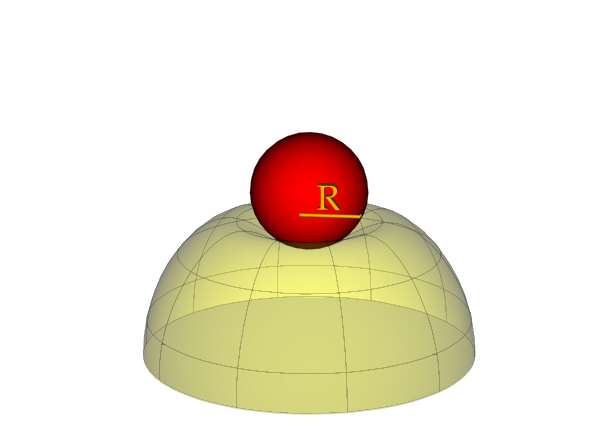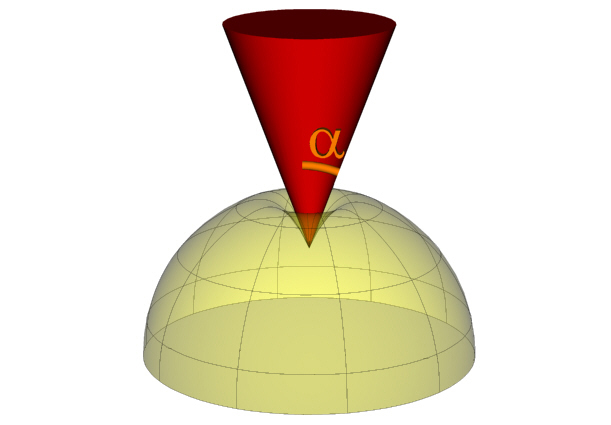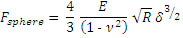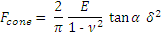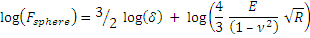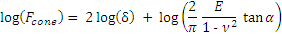which is under the form of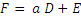with:
 - F the force, - d the sample deformation, - E the Youngs modulus, - n the Poisson ratio, - R the radius of the indenting sphere, - a the opening angle of the indenting cone.

The data obtained in the generated results file are the following:
• file: Name of the experimental file being analyzed.

• power law exponent (-): Power law exponent value of the dependency of the force versus the deformation. This exponent has to be equal to 1.5 (model with sphere) or 2 (model with cone) for the data to be in agreement with the Hertz model.

• intercept (pN.m-ple): Intercept of the curve expressed in pico-Newton per meter exponent minus the value of the power law exponent. It is the value of the intercept obtained by a least square fitting method of the force versus deformation curve in Log-Log representation, that had been reconverted back from the Log form (=10^intercept_value).

• R (-): Pearson product moment correlation coefficient obtained for the power law determination by scanning through the data points between the chosen markers.

• dx (nm): Difference of position in nanometer of the cursor used for the measurements of the sample stiffness.

• dy (pN): Difference of height in pico-Newton of the cursor used for the measurements of the sample stiffness.

• deformation 1 (nm): Deformation in nanometer corresponding to the position of the first cursor used for the measurements of the sample stiffness.

• deformation 2 (nm): Deformation in nanometer corresponding to the position of the second cursor used for the measurements of the sample stiffness.

• height 1 (nm): Height in nanometer of the first cursor used for the measurements of the sample stiffness.

• height 2 (nm): Height in nanometer of the second cursor used for the measurements of the sample stiffness.

• force 1 (pN): Force in pico-Newton of the first cursor used for the measurements of the sample stiffness.

• force 2 (pN): Force in pico-Newton of the second cursor used for the measurements of the sample stiffness.

• involvs (nm/V): Involvs (INVerse Optical LeVer Sensitivity) calibration of the cantilever in nanometer per Volt.

• spring constant (pN/nm): Spring constant value of the cantilever in pico-Newton per nanometer.

• index of baseline (-): Index position of the point used for the baseline.

• index of origin (-): Index position of the point used for the origin of the curve.

A list of the short keys can be found under the Help Menu of Short Keys command.

- Nano-Indentation Fitting [top]
This analysis method can be used in order to analyse nano-indentation type of experiments in order to extract the stiffness of the force versus distance curves.

The data obtained in the generated results file are the following:
• file: Name of the experimental file being analyzed.

• shell radius (um): Radius in micrometer of the shell being analyzed.

• interfacial tension (mN/m): Interfacial tension contribution in milli-Newton per meter of the shell obtained from the fit of the used model.

• membrane stiffness (N/m): Membrane stiffness contribution in Newton per meter of the shell obtained from the fit of the used model.

• R^2 (-): Coefficient of determination obtained for the given fit by scanning through the data points between the chosen markers.

• sample stiffness (pN/nm): Spring constant or stiffness in pico-Newton per nanometer of the measured sample, corresponding to the slope of the force versus deformation curve obtained by a least square fitting method.

• intercept (pN): Intercept in pico-Newton obtained by a least square fitting method of the force versus deformation curve.

• dx (nm): Difference of position in nanometer of the cursor used for the measurements of the sample stiffness.

• dy (pN): Difference of height in pico-Newton of the cursor used for the measurements of the sample stiffness.

• deformation 1 (nm): Deformation in nanometer corresponding to the position of the first cursor used for the measurements of the sample stiffness.

• deformation 2 (nm): Deformation in nanometer corresponding to the position of the second cursor used for the measurements of the sample stiffness.

• height 1 (nm): Height in nanometer of the first cursor used for the measurements of the sample stiffness.

• height 2 (nm): Height in nanometer of the second cursor used for the measurements of the sample stiffness.

• force 1 (pN): Force in pico-Newton of the first cursor used for the measurements of the sample stiffness.

• force 2 (pN): Force in pico-Newton of the second cursor used for the measurements of the sample stiffness.

• involvs (nm/V): Involvs (INVerse Optical LeVer Sensitivity) calibration of the cantilever in nanometer per Volt.

• spring constant (pN/nm): Spring constant value of the cantilever in pico-Newton per nanometer.

• index of baseline (-): Index position of the point used for the baseline.

• index of origin (-): Index position of the point used for the origin of the curve.

A list of the short keys can be found under the Help Menu of Short Keys command.

- Nano-Indentation Hertz Model with Sphere [top]
This analysis method can be used in order to analyse nano-indentation type of experiments with the Hertz model for sphere.
The equation used for performing the curve fitting is the following:with:
 - F the force, - d the sample deformation, - E the Youngs modulus, - n the Poisson ratio, - R the radius of the indenting sphere.

The data obtained in the generated results file are the following:
• file: Name of the experimental file being analyzed.

• Youngs modulus (Pa): Youngs modulus in Pa of the sample being analyzed.

• R^2 (-): Coefficient of determination obtained for the Hertz model with sphere fit by scanning through the data points between the chosen markers.

• Poisson ratio (-): Poisson ratio of the sample being analyzed.

• sphere radius (um): Sphere radius in micron of the probe used on the sample.

• sample stiffness (pN/nm): Spring constant or stiffness in pico-Newton per nanometer of the measured sample, corresponding to the slope of the force versus deformation curve obtained by a least square fitting method.

• intercept (pN): Intercept in pico-Newton obtained by a least square fitting method of the force versus deformation curve.

• dx (nm): Difference of position in nanometer of the cursor used for the measurements of the sample stiffness.

• dy (pN): Difference of height in pico-Newton of the cursor used for the measurements of the sample stiffness.

• deformation 1 (nm): Deformation in nanometer corresponding to the position of the first cursor used for the measurements of the sample stiffness.

• deformation 2 (nm): Deformation in nanometer corresponding to the position of the second cursor used for the measurements of the sample stiffness.

• height 1 (nm): Height in nanometer of the first cursor used for the measurements of the sample stiffness.

• height 2 (nm): Height in nanometer of the second cursor used for the measurements of the sample stiffness.

• force 1 (pN): Force in pico-Newton of the first cursor used for the measurements of the sample stiffness.

• force 2 (pN): Force in pico-Newton of the second cursor used for the measurements of the sample stiffness.

• involvs (nm/V): Involvs (INVerse Optical LeVer Sensitivity) calibration of the cantilever in nanometer per Volt.

• spring constant (pN/nm): Spring constant value of the cantilever in pico-Newton per nanometer.

• index of baseline (-): Index position of the point used for the baseline.

• index of origin (-): Index position of the point used for the origin of the curve.

A list of the short keys can be found under the Help Menu of Short Keys command.

- Nano-Indentation Hertz Model with Cone [top]
This analysis method can be used in order to analyse nano-indentation type of experiments with the Hertz model for cone.
The equation used for performing the curve fitting is the following:with:
 - F the force, - d the sample deformation, - E the Youngs modulus, - n the Poisson ratio, - a the opening angle of the indenting cone.

For more details, see the following paper:
Sneddon I. N.
The relation between load and penetration in the axisymmetric boussinesq problem for a punch of arbitrary profile.
Int. J. Eng. Sci. 1965; 3:47-57.

The data obtained in the generated results file are the following:
• file: Name of the experimental file being analyzed.

• Youngs modulus (Pa): Youngs modulus in Pa of the sample being analyzed.

• R^2 (-): Coefficient of determination obtained for the Hertz model with cone fit by scanning through the data points between the chosen markers.

• Poisson ratio (-): Poisson ratio of the sample being analyzed.

• opening angle (�): Opening angle in degre (�) of the probe used on the sample.

• sample stiffness (pN/nm): Spring constant or stiffness in pico-Newton per nanometer of the measured sample, corresponding to the slope of the force versus deformation curve obtained by a least square fitting method.

• intercept (pN): Intercept in pico-Newton obtained by a least square fitting method of the force versus deformation curve.

• dx (nm): Difference of position in nanometer of the cursor used for the measurements of the sample stiffness.

• dy (pN): Difference of height in pico-Newton of the cursor used for the measurements of the sample stiffness.

• deformation 1 (nm): Deformation in nanometer corresponding to the position of the first cursor used for the measurements of the sample stiffness.

• deformation 2 (nm): Deformation in nanometer corresponding to the position of the second cursor used for the measurements of the sample stiffness.

• height 1 (nm): Height in nanometer of the first cursor used for the measurements of the sample stiffness.

• height 2 (nm): Height in nanometer of the second cursor used for the measurements of the sample stiffness.

• force 1 (pN): Force in pico-Newton of the first cursor used for the measurements of the sample stiffness.

• force 2 (pN): Force in pico-Newton of the second cursor used for the measurements of the sample stiffness.

• involvs (nm/V): Involvs (INVerse Optical LeVer Sensitivity) calibration of the cantilever in nanometer per Volt.

• spring constant (pN/nm): Spring constant value of the cantilever in pico-Newton per nanometer.

• index of baseline (-): Index position of the point used for the baseline.

• index of origin (-): Index position of the point used for the origin of the curve.

A list of the short keys can be found under the Help Menu of Short Keys command.

- Nano-Indentation Hertz Model with Pyramid [top]
This analysis method can be used in order to analyse nano-indentation type of experiments with the Hertz model for pyramid.
The equation used for performing the curve fitting is the following: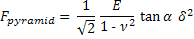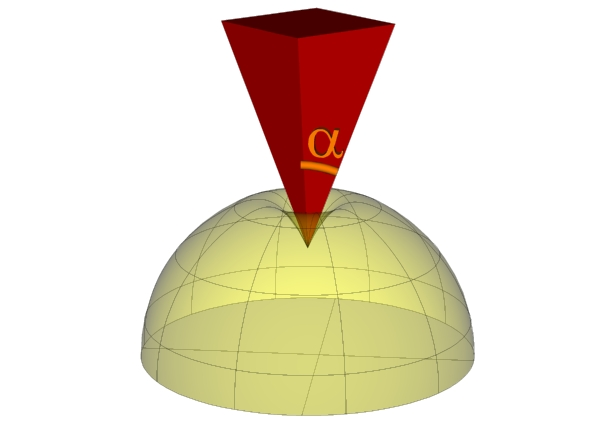with:
 - F the force, - d the sample deformation, - E the Youngs modulus, - n the Poisson ratio, - a the opening angle of the indenting cone.

For more details, see the following paper:
and
The data obtained in the generated results file are the following:
• file: Name of the experimental file being analyzed.

• Youngs modulus (Pa): Youngs modulus in Pa of the sample being analyzed.

• R^2 (-): Coefficient of determination obtained for the Hertz model with cone fit by scanning through the data points between the chosen markers.

• Poisson ratio (-): Poisson ratio of the sample being analyzed.

• opening angle (�): Opening angle in degre (�) of the probe used on the sample.

• sample stiffness (pN/nm): Spring constant or stiffness in pico-Newton per nanometer of the measured sample, corresponding to the slope of the force versus deformation curve obtained by a least square fitting method.

• intercept (pN): Intercept in pico-Newton obtained by a least square fitting method of the force versus deformation curve.

• dx (nm): Difference of position in nanometer of the cursor used for the measurements of the sample stiffness.

• dy (pN): Difference of height in pico-Newton of the cursor used for the measurements of the sample stiffness.

• deformation 1 (nm): Deformation in nanometer corresponding to the position of the first cursor used for the measurements of the sample stiffness.

• deformation 2 (nm): Deformation in nanometer corresponding to the position of the second cursor used for the measurements of the sample stiffness.

• height 1 (nm): Height in nanometer of the first cursor used for the measurements of the sample stiffness.

• height 2 (nm): Height in nanometer of the second cursor used for the measurements of the sample stiffness.

• force 1 (pN): Force in pico-Newton of the first cursor used for the measurements of the sample stiffness.

• force 2 (pN): Force in pico-Newton of the second cursor used for the measurements of the sample stiffness.

• involvs (nm/V): Involvs (INVerse Optical LeVer Sensitivity) calibration of the cantilever in nanometer per Volt.

• spring constant (pN/nm): Spring constant value of the cantilever in pico-Newton per nanometer.

• index of baseline (-): Index position of the point used for the baseline.

• index of origin (-): Index position of the point used for the origin of the curve.

A list of the short keys can be found under the Help Menu of Short Keys command.

- Nano-Indentation Hertz Model Triple [top]
This analysis method can be used in order to analyse nano-indentation type of experiments with the Hertz model triple.
The equation used for performing the curve fitting is the following:with:
 - F the force, - d the sample deformation, - E the Youngs modulus, - n the Poisson ratio, - R the radius of the indenting sphere, - a the opening angle of the indenting cone or pyramid.

The data obtained in the generated results file are the following:
• file: Name of the experimental file being analyzed.

• Youngs modulus sphere (Pa): Youngs modulus in Pa of the sample being analyzed and probed with a sphere indenter.

• Youngs modulus cone (Pa): Youngs modulus in Pa of the sample being analyzed and probed with a cone indenter.

• Youngs modulus pyramid (Pa): Youngs modulus in Pa of the sample being analyzed and probed with a pyramid indenter.

• R^2 sphere (-): Coefficient of determination obtained for the sample being analyzed and probed with a sphere indenter by scanning through the data points between the chosen markers.

• R^2 cone (-): Coefficient of determination obtained for the sample being analyzed and probed with a cone indenter by scanning through the data points between the chosen markers.

• R^2 pyramid (-): Coefficient of determination obtained for the sample being analyzed and probed with a pyramid indenter by scanning through the data points between the chosen markers.

• Poisson ratio (-): Poisson ratio of the sample being analyzed.

• sphere radius (um): Sphere radius in micron of the probe used on the sample.

• sample stiffness (pN/nm): Spring constant or stiffness in pico-Newton per nanometer of the measured sample, corresponding to the slope of the force versus deformation curve obtained by a least square fitting method.

• intercept (pN): Intercept in pico-Newton obtained by a least square fitting method of the force versus deformation curve.

• dx (nm): Difference of position in nanometer of the cursor used for the measurements of the sample stiffness.

• dy (pN): Difference of height in pico-Newton of the cursor used for the measurements of the sample stiffness.

• deformation 1 (nm): Deformation in nanometer corresponding to the position of the first cursor used for the measurements of the sample stiffness.

• deformation 2 (nm): Deformation in nanometer corresponding to the position of the second cursor used for the measurements of the sample stiffness.

• height 1 (nm): Height in nanometer of the first cursor used for the measurements of the sample stiffness.

• height 2 (nm): Height in nanometer of the second cursor used for the measurements of the sample stiffness.

• force 1 (pN): Force in pico-Newton of the first cursor used for the measurements of the sample stiffness.

• force 2 (pN): Force in pico-Newton of the second cursor used for the measurements of the sample stiffness.

• involvs (nm/V): Involvs (INVerse Optical LeVer Sensitivity) calibration of the cantilever in nanometer per Volt.

• spring constant (pN/nm): Spring constant value of the cantilever in pico-Newton per nanometer.

• index of baseline (-): Index position of the point used for the baseline.

• index of origin (-): Index position of the point used for the origin of the curve.

A list of the short keys can be found under the Help Menu of Short Keys command.

- Nano-Indentation Hertz Model Triple with Adhesion [top]
This analysis method can be used in order to analyse nano-indentation type of experiments with the Hertz model triple with adhesion.
The equation used for performing the curve fitting is the following: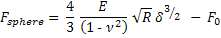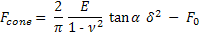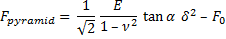with:
 - F the force, - d the sample deformation, - E the Youngs modulus, - n the Poisson ratio, - R the radius of the indenting sphere, - a the opening angle of the indenting cone or pyramid, - F0 the adhesion force.

The data obtained in the generated results file are the following:
• file: Name of the experimental file being analyzed.

• Youngs modulus sphere (Pa): Youngs modulus in Pa of the sample being analyzed and probed with a sphere indenter.

• Youngs modulus cone (Pa): Youngs modulus in Pa of the sample being analyzed and probed with a cone indenter.

• Youngs modulus pyramid (Pa): Youngs modulus in Pa of the sample being analyzed and probed with a pyramid indenter.

• R^2 sphere (-): Coefficient of determination obtained for the sample being analyzed and probed with a sphere indenter by scanning through the data points between the chosen markers.

• R^2 cone (-): Coefficient of determination obtained for the sample being analyzed and probed with a cone indenter by scanning through the data points between the chosen markers.

• R^2 pyramid (-): Coefficient of determination obtained for the sample being analyzed and probed with a pyramid indenter by scanning through the data points between the chosen markers.

• Poisson ratio (-): Poisson ratio of the sample being analyzed.

• sphere radius (um): Sphere radius in micron of the probe used on the sample.

• adhesion force sphere (pN): Adhesion force in pico-Newton of the sample being analyzed and probed with a sphere indenter.

• adhesion force cone (pN): Adhesion force in pico-Newton of the sample being analyzed and probed with a cone indenter.

• adhesion force pyramid (pN): Adhesion force in pico-Newton of the sample being analyzed and probed with a pyramid indenter.

• adhesion energy (pN.nm): Adhesion energy in pico-Newton nanometer of the sample being analyzed.

• sample stiffness (pN/nm): Spring constant or stiffness in pico-Newton per nanometer of the measured sample, corresponding to the slope of the force versus deformation curve obtained by a least square fitting method.

• intercept (pN): Intercept in pico-Newton obtained by a least square fitting method of the force versus deformation curve.

• dx (nm): Difference of position in nanometer of the cursor used for the measurements of the sample stiffness.

• dy (pN): Difference of height in pico-Newton of the cursor used for the measurements of the sample stiffness.

• deformation 1 (nm): Deformation in nanometer corresponding to the position of the first cursor used for the measurements of the sample stiffness.

• deformation 2 (nm): Deformation in nanometer corresponding to the position of the second cursor used for the measurements of the sample stiffness.

• height 1 (nm): Height in nanometer of the first cursor used for the measurements of the sample stiffness.

• height 2 (nm): Height in nanometer of the second cursor used for the measurements of the sample stiffness.

• force 1 (pN): Force in pico-Newton of the first cursor used for the measurements of the sample stiffness.

• force 2 (pN): Force in pico-Newton of the second cursor used for the measurements of the sample stiffness.

• curves difference area (pN.nm): Curves difference area in Pico Newton nanometers, corresponding to the area found between the extension and retraction curve.

• involvs (nm/V): Involvs (INVerse Optical LeVer Sensitivity) calibration of the cantilever in nanometer per Volt.

• spring constant (pN/nm): Spring constant value of the cantilever in pico-Newton per nanometer.

• index of baseline (-): Index position of the point used for the baseline.

• index of origin (-): Index position of the point used for the origin of the curve.

• index of area (-): Index position of the first marker used for the adhesion energy analysis.

• index of difference (-): Index position of the first marker used for the curve difference area analysis.

A list of the short keys can be found under the Help Menu of Short Keys command.

- Nano-Indentation Linear Hertz Model with Sphere [top]
This analysis method can be used in order to analyse nano-indentation type of experiments with the linear Hertz model for sphere.
The Youngs modulus is calculated in the following way: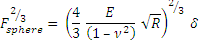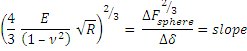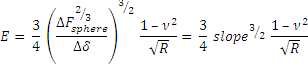with:
 - F the force, - d the sample deformation, - E the Youngs modulus, - n the Poisson ratio, - R the radius of the indenting sphere.

For more details, see the following paper:

The data obtained in the generated results file are the following:
• file: Name of the experimental file being analyzed.

• Youngs modulus (Pa): Youngs modulus in Pa of the sample being analyzed.

• R (-): Pearson product moment correlation coefficient obtained for the exponent linear fit determination by scanning through the data points between the chosen markers.

• Poisson ratio (-): Poisson ratio of the sample being analyzed.

• sphere radius (um): Sphere radius in micron of the probe used on the sample.

• dx (nm): Difference of position in nanometer of the cursor used for the measurements of the sample stiffness.

• dy (pN^2/3): Difference of height in pico-Newton power two third (pN2/3) of the cursor used for the measurements of the sample stiffness.

• deformation 1 (nm): Deformation in nanometer corresponding to the position of the first cursor used for the measurements of the sample stiffness.

• deformation 2 (nm): Deformation in nanometer corresponding to the position of the second cursor used for the measurements of the sample stiffness.

• force 1 (pN^2/3): Force in pico-Newton power two third (pN2/3) of the first cursor used for the measurements of the sample stiffness.

• force 2 (pN^2/3): Force in pico-Newton power two third (pN2/3) of the second cursor used for the measurements of the sample stiffness.

• involvs (nm/V): Involvs (INVerse Optical LeVer Sensitivity) calibration of the cantilever in nanometer per Volt.

• spring constant (pN/nm): Spring constant value of the cantilever in pico-Newton per nanometer.

• index of baseline (-): Index position of the point used for the baseline.

• index of origin (-): Index position of the point used for the origin of the curve.

A list of the short keys can be found under the Help Menu of Short Keys command.

- Nano-Indentation linear Hertz Model with Cone [top]
This analysis method can be used in order to analyse nano-indentation type of experiments with the linear Hertz model for cone.
The Youngs modulus is calculated in the following way: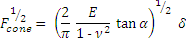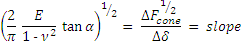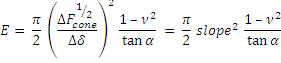with:
 - F the force, - d the sample deformation, - E the Youngs modulus, - n the Poisson ratio, - a the opening angle of the indenting cone.

For more details, see the following paper:

The data obtained in the generated results file are the following:
• file: Name of the experimental file being analyzed.

• Youngs modulus (Pa): Youngs modulus in Pa of the sample being analyzed.

• R (-): Pearson product moment correlation coefficient obtained for the exponent linear fit determination by scanning through the data points between the chosen markers.

• Poisson ratio (-): Poisson ratio of the sample being analyzed.

• opening angle (�): Opening angle in degre (�) of the probe used on the sample.

• dx (nm): Difference of position in nanometer of the cursor used for the measurements of the sample stiffness.

• dy (pN^1/2): Difference of height in pico-Newton power one half (pN1/2) of the cursor used for the measurements of the sample stiffness.

• deformation 1 (nm): Deformation in nanometer corresponding to the position of the first cursor used for the measurements of the sample stiffness.

• deformation 2 (nm): Deformation in nanometer corresponding to the position of the second cursor used for the measurements of the sample stiffness.

• force 1 (pN^1/2): Force in pico-Newton power one half (pN1/2) of the first cursor used for the measurements of the sample stiffness.

• force 2 (pN^1/2): Force in pico-Newton power one half (pN1/2) of the second cursor used for the measurements of the sample stiffness.

• involvs (nm/V): Involvs (INVerse Optical LeVer Sensitivity) calibration of the cantilever in nanometer per Volt.

• spring constant (pN/nm): Spring constant value of the cantilever in pico-Newton per nanometer.

• index of baseline (-): Index position of the point used for the baseline.

• index of origin (-): Index position of the point used for the origin of the curve.

A list of the short keys can be found under the Help Menu of Short Keys command.

- Nano-Indentation Hertz Model for Layer with Sphere [top]
This analysis method can be used in order to analyse nano-indentation type of experiments with the Hertz model for layer for sphere.
The equations used for performing the curve fitting are taken from the following paper:

Where: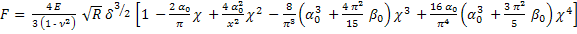with: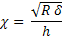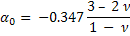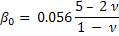and:
 - F the force, - d the sample deformation, - E the Youngs modulus, - n the Poisson ratio, - R the radius of the indenting sphere.

The data obtained in the generated results file are the following:
• file: Name of the experimental file being analyzed.

• Youngs modulus (Pa): Youngs modulus in Pa of the sample being analyzed.

• R^2 (-): Coefficient of determination obtained for the Hertz model with sphere fit by scanning through the data points between the chosen markers.

• Poisson ratio (-): Poisson ratio of the sample being analyzed.

• sphere radius (um): Sphere radius in micron of the probe used on the sample.

• layer thicknes (nm): Thickness in nm of the sample layer.

• sample stiffness (pN/nm): Spring constant or stiffness in pico-Newton per nanometer of the measured sample, corresponding to the slope of the force versus deformation curve obtained by a least square fitting method.

• intercept (pN): Intercept in pico-Newton obtained by a least square fitting method of the force versus deformation curve.

• dx (nm): Difference of position in nanometer of the cursor used for the measurements of the sample stiffness.

• dy (pN): Difference of height in pico-Newton of the cursor used for the measurements of the sample stiffness.

• deformation 1 (nm): Deformation in nanometer corresponding to the position of the first cursor used for the measurements of the sample stiffness.

• deformation 2 (nm): Deformation in nanometer corresponding to the position of the second cursor used for the measurements of the sample stiffness.

• height 1 (nm): Height in nanometer of the first cursor used for the measurements of the sample stiffness.

• height 2 (nm): Height in nanometer of the second cursor used for the measurements of the sample stiffness.

• force 1 (pN): Force in pico-Newton of the first cursor used for the measurements of the sample stiffness.

• force 2 (pN): Force in pico-Newton of the second cursor used for the measurements of the sample stiffness.

• involvs (nm/V): Involvs (INVerse Optical LeVer Sensitivity) calibration of the cantilever in nanometer per Volt.

• spring constant (pN/nm): Spring constant value of the cantilever in pico-Newton per nanometer.

• index of baseline (-): Index position of the point used for the baseline.

• index of origin (-): Index position of the point used for the origin of the curve.

A list of the short keys can be found under the Help Menu of Short Keys command.

- Nano-Indentation Oliver and Pharr [top]
This analysis method can be used in order to analyse nano-indentation type of experiments with the Hertz model for cone.
The equation used for calculating the Young's modulus is the following: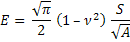and for the hardness: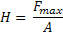with:
 - E the Youngs modulus, - H the Hardness, - n the Poisson ratio, - S the contact stiffness, - A the area function, - Fmax the maximum force.

with S defined as: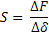with:
 - F the force, - d the sample deformation,

and: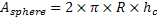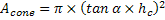with:
 - R the radius of the indenting sphere, - a the opening angle of the indenting cone, - hC the contact depth.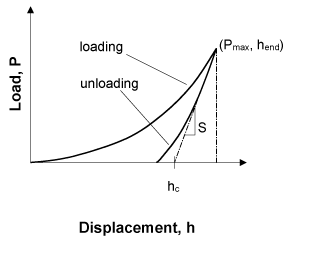The data obtained in the generated results file are the following:
• file: Name of the experimental file being analyzed.

• Youngs modulus sphere (Pa): Youngs modulus in Pa of the sample being analyzed by using a sphere indenter.

• Youngs modulus cone (Pa): Youngs modulus in Pa of the sample being analyzed by using a cone indenter.

• Hardness sphere (Pa): Hardness in Pa of the sample being analyzed by using a sphere indenter.

• Hardness cone (Pa): Hardness in Pa of the sample being analyzed by using a cone indenter.

• sample stiffness (pN/nm): Spring constant or stiffness in pico-Newton per nanometer of the measured sample, corresponding to the slope of the force versus deformation curve obtained by a least square fitting method.

• inverse sample stiffness (nm/pN): Inverse spring constant or stiffness in nanometer per pico-Newton of the measured sample, corresponding to the inverse of the slope of the force versus deformation curve obtained by a least square fitting method (= 1 / sample stiffness (pN/nm)). These values can easily be used in order to calculate an average sensibility value by setting the INVOLVS value to 1 nm/V as well as the Canteliver Spring Constant value to 1 pN/nm.

• intercept (pN): Intercept in pico-Newton obtained by a least square fitting method of the force versus deformation curve.

• R (-): Pearson product moment correlation coefficient obtained for the sample stiffness determination by scanning through the data points between the chosen markers.

• Poisson ratio (-): Poisson ratio of the sample being analyzed.

• sphere radius (um): Sphere radius in micron of the probe used on the sample.

• opening angle (�): Opening angle in degre (�) of the probe used on the sample.

• dx (nm): Difference of position in nanometer of the cursor used for the measurements of the sample stiffness.

• dy (pN): Difference of height in pico-Newton of the cursor used for the measurements of the sample stiffness.

• deformation 1 (nm): Deformation in nanometer corresponding to the position of the first cursor used for the measurements of the sample stiffness.

• deformation 2 (nm): Deformation in nanometer corresponding to the position of the second cursor used for the measurements of the sample stiffness.

• height 1 (nm): Height in nanometer of the first cursor used for the measurements of the sample stiffness.

• height 2 (nm): Height in nanometer of the second cursor used for the measurements of the sample stiffness.

• force 1 (pN): Force in pico-Newton of the first cursor used for the measurements of the sample stiffness.

• force 2 (pN): Force in pico-Newton of the second cursor used for the measurements of the sample stiffness.

• involvs (nm/V): Involvs (INVerse Optical LeVer Sensitivity) calibration of the cantilever in nanometer per Volt.

• spring constant (pN/nm): Spring constant value of the cantilever in pico-Newton per nanometer.

• index of baseline (-): Index position of the point used for the baseline.

• index of origin (-): Index position of the point used for the origin of the curve.

A list of the short keys can be found under the Help Menu of Short Keys command.

- Nano-Indentation JKR [top]
This analysis method can be used in order to analyse nano-indentation type of experiments with the JKR (Johnson, Kendall and Roberts) model.
The JKR theory is expressed by the following two equations: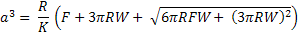with:
 - F the force, - d the sample deformation, - a the contact radius, - R the tip radius, - W the adhesion energy, - K the combined elastic modulus given by the following relation,

and: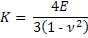with:
 - E the Youngs modulus, - n the Poisson ratio.

Using the two point method, the JKR equations are converted to the following functions where K and W are explicitly presented: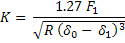For more details, see the following papers:
and
The data obtained in the generated results file are the following:
• file: Name of the experimental file being analyzed.

• Youngs modulus (Pa): Youngs modulus in Pa of the sample being analyzed.

• R^2 (-): Coefficient of determination obtained for the Hertz model with sphere fit by scanning through the data points between the chosen markers.

• Poisson ratio (-): Poisson ratio of the sample being analyzed.

• sphere radius (um): Sphere radius in micron of the probe used on the sample.

• adhesion force (pN): Adhesion force in pico-Newton of the sample being analyzed.

• adhesion energy (pN.nm): Adhesion energy in pico-Newton nanometer of the sample being analyzed.

• adhesion work (pN/nm): Adhesion work in pico-Newton per nanometer of the sample being analyzed.

• sample stiffness (pN/nm): Spring constant or stiffness in pico-Newton per nanometer of the measured sample, corresponding to the slope of the force versus deformation curve obtained by a least square fitting method.

• intercept (pN): Intercept in pico-Newton obtained by a least square fitting method of the force versus deformation curve.

• dx (nm): Difference of position in nanometer of the cursor used for the measurements of the sample stiffness.

• dy (pN): Difference of height in pico-Newton of the cursor used for the measurements of the sample stiffness.

• deformation 1 (nm): Deformation in nanometer corresponding to the position of the first cursor used for the measurements of the sample stiffness.

• deformation 2 (nm): Deformation in nanometer corresponding to the position of the second cursor used for the measurements of the sample stiffness.

• height 1 (nm): Height in nanometer of the first cursor used for the measurements of the sample stiffness.

• height 2 (nm): Height in nanometer of the second cursor used for the measurements of the sample stiffness.

• force 1 (pN): Force in pico-Newton of the first cursor used for the measurements of the sample stiffness.

• force 2 (pN): Force in pico-Newton of the second cursor used for the measurements of the sample stiffness.

• curves difference area (pN.nm): Curves difference area in Pico Newton nanometers, corresponding to the area found between the extension and retraction curve.

• involvs (nm/V): Involvs (INVerse Optical LeVer Sensitivity) calibration of the cantilever in nanometer per Volt.

• spring constant (pN/nm): Spring constant value of the cantilever in pico-Newton per nanometer.

• index of baseline (-): Index position of the point used for the baseline.

• index of origin (-): Index position of the point used for the origin of the curve.

• index of area (-): Index position of the first marker used for the adhesion energy analysis.

• index of difference (-): Index position of the first marker used for the curve difference area analysis.

A list of the short keys can be found under the Help Menu of Short Keys command.

- Nano-Indentation DMT [top]
This analysis method can be used in order to analyse nano-indentation type of experiments with the DMT (Derjaguin, Muller and Toporov) model.
The equation used for performing the curve fitting are the following: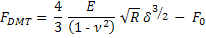with:
 - F the force, - d the sample deformation, - E the Youngs modulus, - n the Poisson ratio, - R the radius of the indenting sphere, - F0 the adhesion force,

and: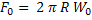with:
 - W0 the adhesion work.

The data obtained in the generated results file are the following:
• file: Name of the experimental file being analyzed.

• Youngs modulus (Pa): Youngs modulus in Pa of the sample being analyzed.

• R^2 (-): Coefficient of determination obtained for the Hertz model with sphere fit by scanning through the data points between the chosen markers.

• Poisson ratio (-): Poisson ratio of the sample being analyzed.

• sphere radius (um): Sphere radius in micron of the probe used on the sample.

• adhesion force (pN): Adhesion force in pico-Newton of the sample being analyzed.

• adhesion energy (pN.nm): Adhesion energy in pico-Newton nanometer of the sample being analyzed.

• adhesion work (pN/nm): Adhesion work in pico-Newton per nanometer of the sample being analyzed.

• sample stiffness (pN/nm): Spring constant or stiffness in pico-Newton per nanometer of the measured sample, corresponding to the slope of the force versus deformation curve obtained by a least square fitting method.

• intercept (pN): Intercept in pico-Newton obtained by a least square fitting method of the force versus deformation curve.

• dx (nm): Difference of position in nanometer of the cursor used for the measurements of the sample stiffness.

• dy (pN): Difference of height in pico-Newton of the cursor used for the measurements of the sample stiffness.

• deformation 1 (nm): Deformation in nanometer corresponding to the position of the first cursor used for the measurements of the sample stiffness.

• deformation 2 (nm): Deformation in nanometer corresponding to the position of the second cursor used for the measurements of the sample stiffness.

• height 1 (nm): Height in nanometer of the first cursor used for the measurements of the sample stiffness.

• height 2 (nm): Height in nanometer of the second cursor used for the measurements of the sample stiffness.

• force 1 (pN): Force in pico-Newton of the first cursor used for the measurements of the sample stiffness.

• force 2 (pN): Force in pico-Newton of the second cursor used for the measurements of the sample stiffness.

• curves difference area (pN.nm): Curves difference area in Pico Newton nanometers, corresponding to the area found between the extension and retraction curve.

• involvs (nm/V): Involvs (INVerse Optical LeVer Sensitivity) calibration of the cantilever in nanometer per Volt.

• spring constant (pN/nm): Spring constant value of the cantilever in pico-Newton per nanometer.

• index of baseline (-): Index position of the point used for the baseline.

• index of origin (-): Index position of the point used for the origin of the curve.

• index of area (-): Index position of the first marker used for the adhesion energy analysis.

• index of difference (-): Index position of the first marker used for the curve difference area analysis.

A list of the short keys can be found under the Help Menu of Short Keys command.

• How can I know which short key I need to press in order to perform a given process? [top]
• By selecting 'Help->Help of short keys' or 'F2' you get a list of all the available short keys and their function in the given type of analysis.
• You can also get a list of the short keys by selecting 'Help->Help Topics' (or 'F3').
Then select 'Menus->Help menu->Help Menu of Short Keys' and finally the type of analysis for which you would like to get the short keys help.

• The content of the Help Menu of Short Keys will be different depending on the type of analysis:
 Protein Unfolding Protein Unfolding WLCM Protein Unfolding FJCM Protein Unfolding FRCM Force Steps Surface Breakthrough Nano-Indentation Nano-Indentation Log Nano-Indentation Fitting Nano-Indentation Hertz Model with sphere Nano-Indentation Hertz Model with cone Nano-Indentation Hertz Model with pyramid Nano-Indentation Hertz Model triple Nano-Indentation Hertz Model triple with adhesion Nano-Indentation Linear Hertz Model with Sphere Nano-Indentation Linear Hertz Model with Cone Nano-Indentation Hertz Model for Layer with sphere Nano-Indentation Oliver and Pharr Nano-Indentation JKR or Nano-Indentation DMT
you are performing.

• I installed PUNIAS on my machine and tried to use it but it always gives me the following error message: [top]
"You refused the conditions of use of PUNIAS
For any questions or purposes, please contact technical support at:
punias@free.fr
This program will now terminate!!!"
• The first time you are running PUNIAS, it displays a window where it asks you to either accept or decline the conditions of use.
In the case you press the accept button a file named "PUNIAS.ini" will be created either in the software, "c:\winnt" or "c:\windows" folder.
This file contains all the configuration data that the program needs (type of analysis, type of zoom, display of raw curves,...) and each time the user changes one of this configuration options by using the software, this file is rewritten saving the new options.
This has the advantage to save the configurations the users like and he doesn't need to for example choose the type of analysis he wants to perform at each new startup of the software.
But if you refuse the conditions of use or even try to close the condition of use window this file will still saved.
But the information stored won't allow the user to use the software anymore and a window with "Refused conditions of use" will only be displayed.
In order to fix this problem you just need to erase the "PUNIAS.ini" file, start again the software and accept the conditions of use.

• Why is PUNIAS not anymore distributed as a freeware like it has been for several years? [top]
• PUNIAS started to be developed for now almost 10 years ago.

• PUNIAS has been distributed as a freeware for more than 5 years, and thus has reached a certain maturity.

• At last, but not at least, some commercial software used the fact that PUNIAS was free access software in order to copy it.
And thus try to make money from the work and ideas of the authors, who were giving everything to the community for free.

### Answers about nano-indentation

• Should I rather use a colloidal probe or regular (i.e. with sharp tip) cantilever for performing my indentation experiences? [top]
• All depends what kind of information you want to extract for your experiences.
• Is it the average mechanical properties of your objects (i.e. biological cells, emulsion droplets,...)?
Then some colloidal probes cantilevers should be used.
• Is it rather the sub-micrometer mechanical properties of your objects?
Then a regular cantilever with a sharp tip should rather be used.

• The colloidal probe cantilever avoids any injury to biological cells which could be induced by using a sharp tip.

• The colloidal probe cantilever provides a defined and reproducible geometry for performing the experiences.

• Why should I calibrate the sensitivity of the cantilever? [top]
• All what an AFM facility measures is the deflection value measured (in V) as the difference of potential between two quadrants of the photo-detectors.

• But the quantity that is needed to be calculated is a force that is the product of the deflection (in V) with the sensitivity (nm/V) and the spring constant of the cantilever (pN/nm).

• Thus the sensitivity (= INVOLVS: INVerse Optical Lever Sensitivity) relates the deflection in V to a deflection in nm and its calibration is ABSOLUTELY NEEDED for quantitative force spectroscopy data acquisitions.

• The sensitivity is actually a calibration of the laser position on the cantilever and thus once calibrated the laser position on the cantilever should not be modified before finishing the acquisition of the force spectroscopy data.

• The best way to validate the measurements is to measure the sensitivity prior and after the force spectroscopy acquisitions and to check whether these values lie within 10% of error.

• Why should I calibrate the spring constant of the cantilever? [top]
• The spring constant (pN/nm) of the cantilever is needed to transform the measured deflection (already transformed in nm; see previous question on how to make this transformation) into a force.

• It may indeed be possible to just take the nominal spring constant value given by the constructor as the value used in the calculations.
Nevertheless, the spring constant value of a cantilever is given by the following equation: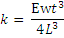with:
 - E the Youngs or elastic modulus modulus of the cantilever's material, - w the cantilever's width, - t the cantilever's thickness, - L the cantilever's Length.

But as the length and width of a cantilever can reproducibly be produced, it is way more difficult to reproducibly produce cantilevers with identical thickness. And as the spring constant value depends on the power 3 with the thickness, some slight changes in the thickness of a cantilever will induce some big variations in the actual spring constant value. This is why it is highly recommended to calibrate the spring constant value of a cantilever and not just use the nominal value.

• But knowing that the resonnance frequency of a cantilever fn varies linearly with the cantilever thickness t through the following equation: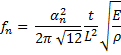with:
 - fn resonnance frequencey of a mode number n of a cantilever, - an the solution of a set of flexural vibration modes of mode number n, - t the cantilever's thickness, - L the cantilever's Length. - E the Youngs or elastic modulus modulus of the cantilever's material, - r the mass density. for more details, see equation 6 of: K Babaei Gavan, E W J M van der Drift, W J Venstra, M R Zuiddam and H S J van der Zant Effect of undercut on the resonant behaviour of silicon nitride cantilevers J. Micromechanics Microengineering 2009; 19 035003

we can conclude that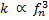and thus using a rule of three, we can calculate the spring constant using:

 Nominal spring constant N/m Nominal frequency kHz Measured frequency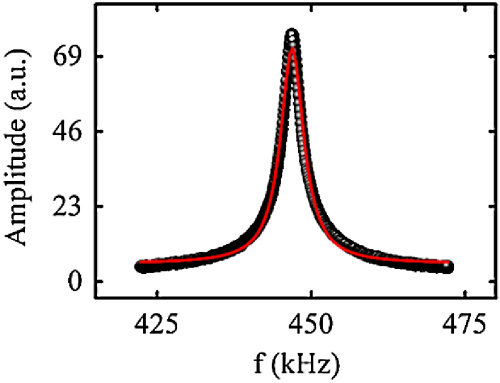kHz kHz kHz kHz kHz Calculated spring constant N/m

• How can I transform the piezo displacement given by my AFM facility during a measurement into deformation or extension? [top]
• The transformation requires the use of a calibrated Piezo in order to correctly measure the displacement.

• The deformation of a sample or extension of a chain is obtained by substracting the deflection [m] from the piezo displacement [m]:
Deformation [m] = Piezo displacement [m] - Deflection [m]
or
Extension [m] = Piezo displacement [m] - Deflection [m]Determine the cantilever sensitivity in order to transform a deflection in V into a deflection in m (or nm): Deflection [m] = Deflection [V] � sensitivity [m/V] (1)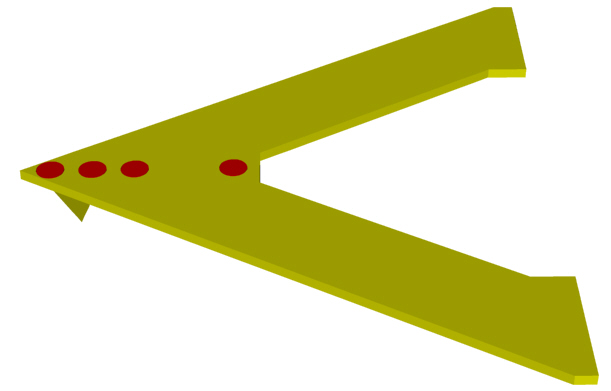Determine the spring constant in order to transform a deflection in m into a force in N (or pN): Force [N] = Deflection [m] � spring constant [N/m] (2)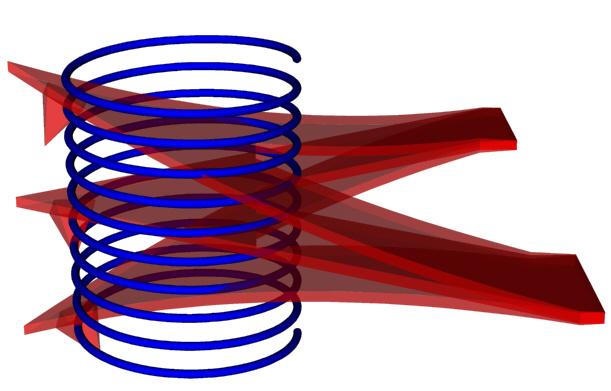Thus the combinaison of the equations (1) and (2) gives: Force [N] = Deflection [V] � sensitivity [m/V] � spring constant [N/m] (3)

• What is the meaning of the following physical quantities: [top]
• Stiffness
•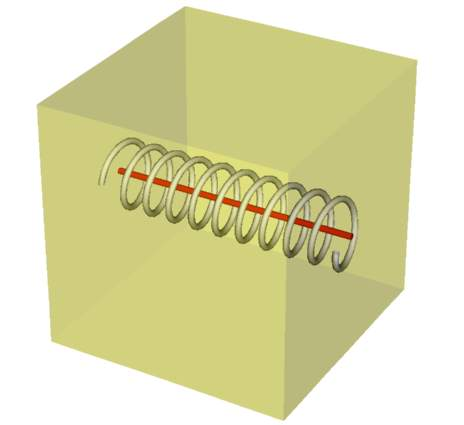The stiffness is an extrinsic property. It represents the spring within a material. It's unit is thus similar to the one of a spring constant, i.e. [N/m].
• Young's modulus
•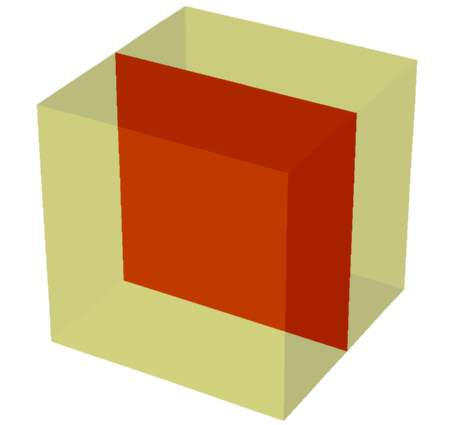The Young's modulus is an intrinsic property. It represents the spring of a material but with a defined contact geometry, i.e. takes the test area into account. It's unit is similar to the one of a pressure, i.e. [Pa], [N.m-2] or [m-1�kg�s-2].
• Poisson's ratio
•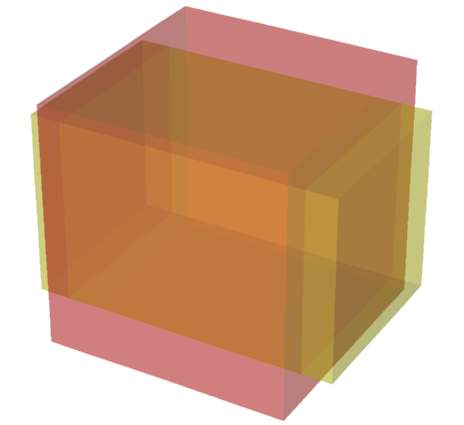The Poisson's ratio is the transverse expansion or contraction of material. The Poisson's ratio is unitles [-].

• I have decided to use the Hertz model in order to analyze my indentation data acquired with a regular (= sharp) tip.
But should I rather use the Hertz model for the sphere or the cone?
[top]
• The equation for the Hertz model of the sphere is:and for the Hertz model of the cone:with E the Young's modulus, ν the Poisson's ratio, R the radius of the sphere and α the half opening angle of the cone.
• By applying a log-log transformation on both sides of the equation, we obtain for the model of the sphere the following equation:and for the model of the cone:• Which is an equation in the form:• Thus by measuring the slope of the force curve represented with the log deformation versus the log of the force, it is possible to figure out which model (between the model of the sphere or cone).

• I have decided to use the Hertz model in order to analyze my indentation data.
But I have really some problems for determining the position of the origin of the curve. Is there a way to simplify or get rid of the curve origin determination?
[top]
• The equation for the Hertz model of the sphere is:and for the Hertz model of the cone:with E the Young's modulus, ν the Poisson's ratio, R the radius of the sphere and α the half opening angle of the cone.
• By linearizing the equations with respect to the deformation δ, we obtain for the model of the sphere the following equation:and for the model of the cone:• By calculating a difference at two deformation positions, we obtain for the model of the sphere the following equation:and for the model of the cone:And this difference operation at two different deformation positions gets simultaneously rid of the origin position (i.e. there is not anymore the need to determine it).
• And from the previous equations, the Young's modulus calculation for the model of the sphere gives the following equation:and for the model of the cone:Thus by representing the force curves as force at the power 2/3 in the case of the model of the sphere and force at the power 1/2 versus the deformation, it is possible to get rid of the determination of the origin position by getting the difference at two positions: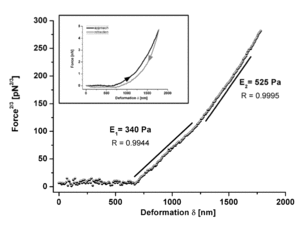For more detailed information see: